# Python机器学习笔记：sklearn库的学习

网上有很多关于sklearn的学习教程，大部分都是简单的讲清楚某一方面，所以最好的教程其实就是官方文档。

（可是官方文档非常详细，同时许多人对官方文档的理解和结构上都不能很好地把握，我也打算好好学习sklearn，这可能是机器学习的神器），下面先简单介绍一下sklearn。

自2007年发布以来，scikit-learn已经成为Python重要的机器学习库了，scikit-learn简称sklearn，支持包括分类，回归，降维和聚类四大机器学习算法。还包括了特征提取，数据处理和模型评估者三大模块。

sklearn是Scipy的扩展，建立在Numpy和matplolib库的基础上。利用这几大模块的优势，可以大大的提高机器学习的效率。

sklearn拥有着完善的文档，上手容易，具有着丰富的API，在学术界颇受欢迎。sklearn已经封装了大量的机器学习算法，包括LIBSVM和LIBINEAR。同时sklearn内置了大量数据集，节省了获取和整理数据集的时间。

## 一，sklearn官方文档的内容和结构

### 1.1 sklearn官方文档的内容

定义：针对经验E和一系列的任务T和一定表现的衡量P，如果随着经验E的积累，针对定义好的任务T可以提高表现P，就说明机器具有学习能力。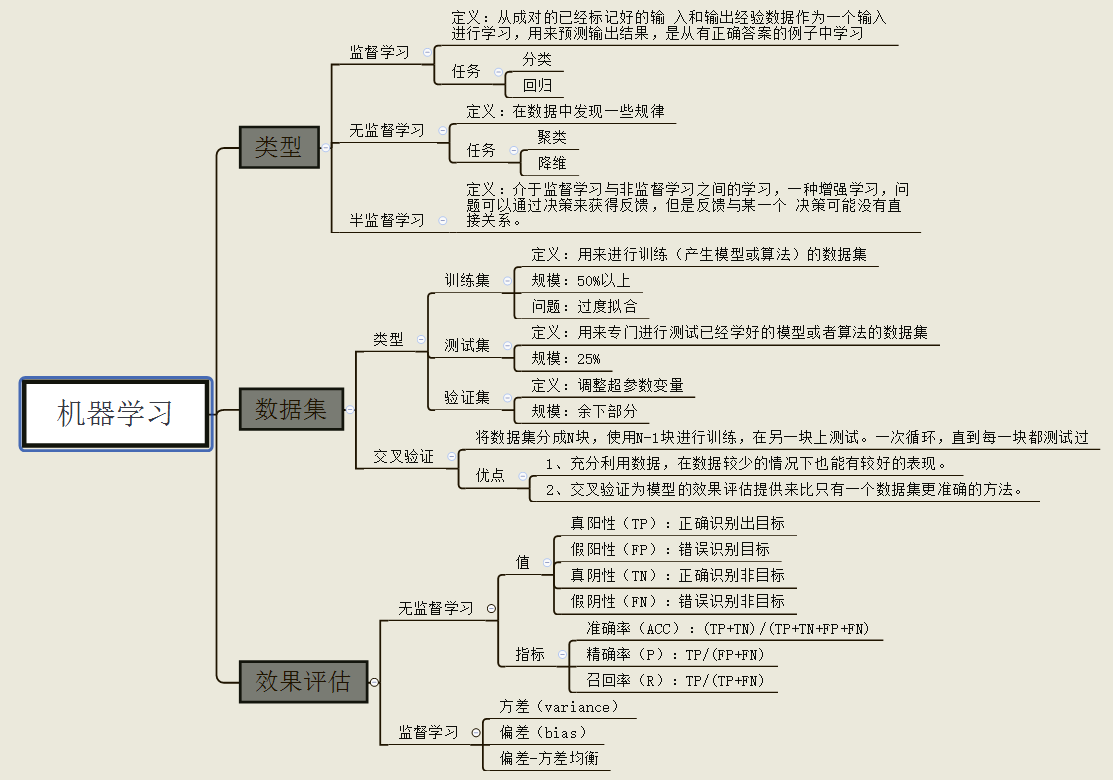### 1.2 sklearn官方文档结构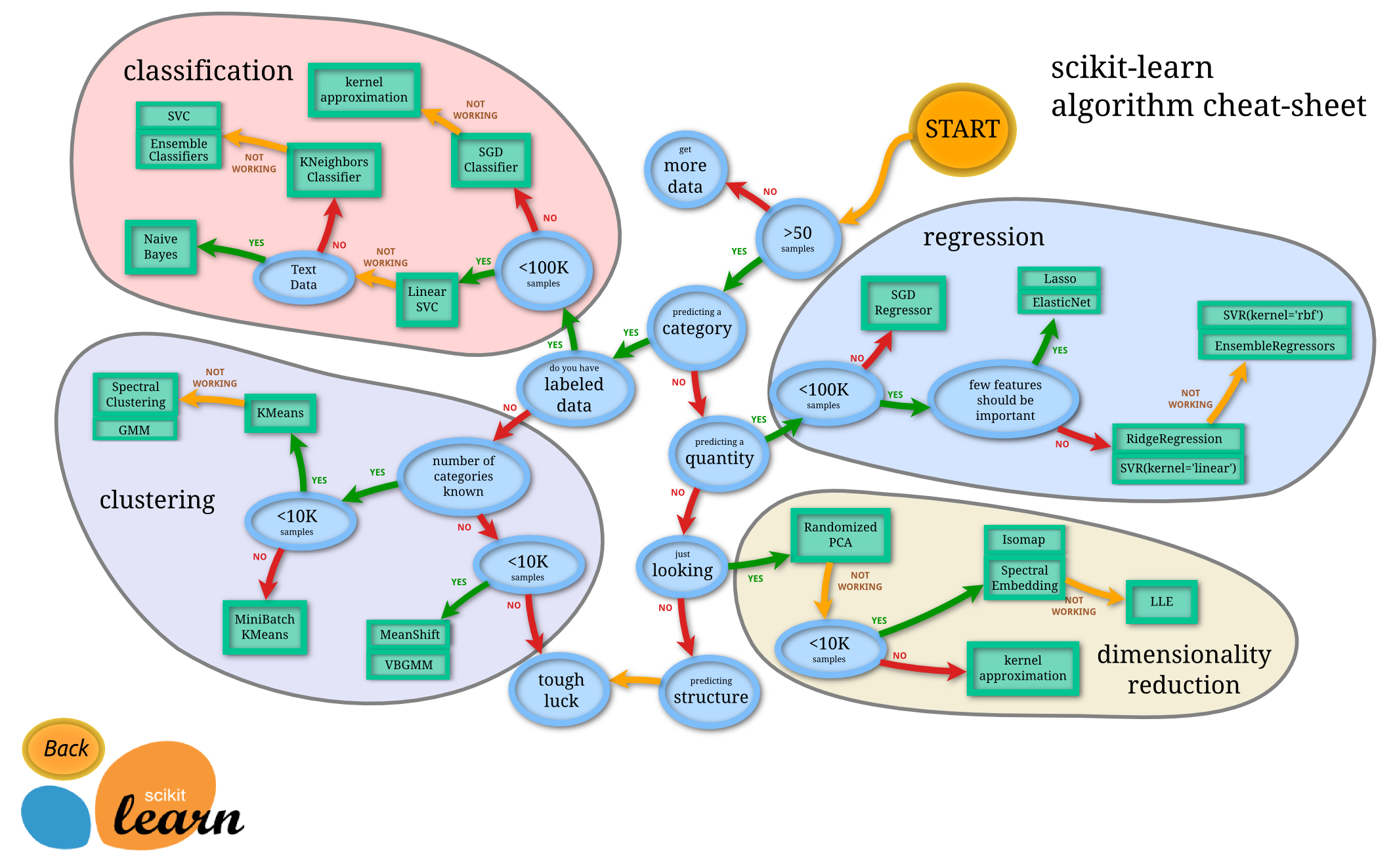由图中，可以看到库的算法主要有四类：分类，回归，聚类，降维。其中：

• 常用聚类：k均值（K-means）、层次聚类（Hierarchical clustering）、DBSCAN
• 常用降维：LinearDiscriminantAnalysis、PCA

这个流程图代表：蓝色圆圈是判断条件，绿色方框是可以选择的算法，我们可以根据自己的数据特征和任务目标去找一条自己的操作路线。

sklearn中包含众多数据预处理和特征工程相关的模块，虽然刚接触sklearn时，大家都会为其中包含的各种算法的广度深度所震惊，但其实sklearn六大板块中有两块都是关于数据预处理和特征工程的，两个板块互相交互，为建模之前的全部工程打下基础。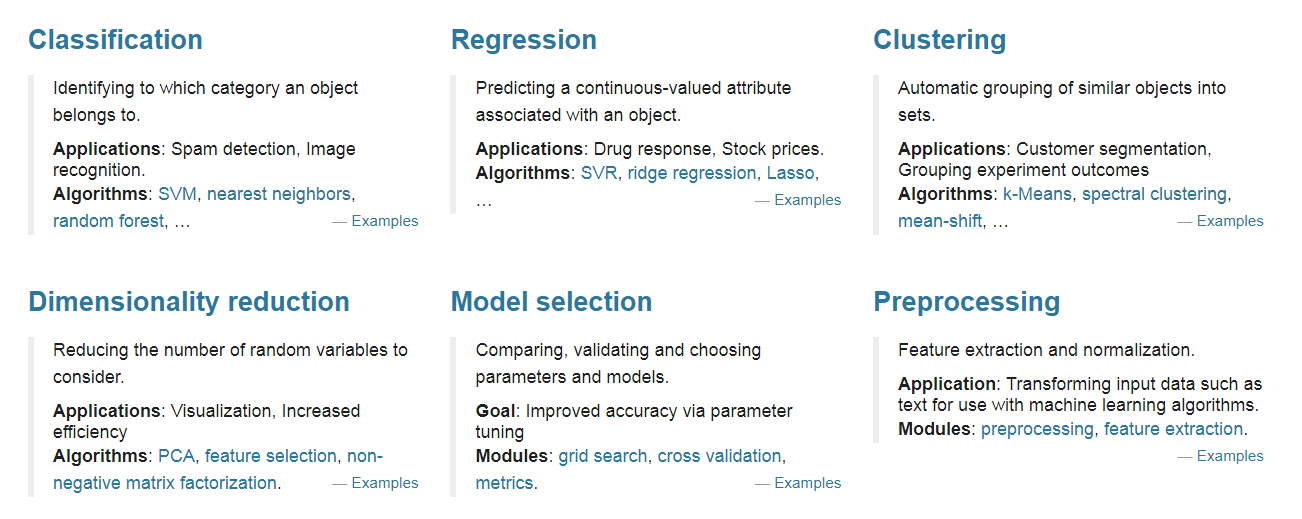• 模块preprocessing：几乎包含数据预处理的所有内容
• 模块Impute：填补缺失值专用
• 模块feature_selection：包含特征选择的各种方法的实践
• 模块decomposition：包含降维算法

## 二，sklearn的快速使用

传统的机器学习任务从开始到建模的一般流程就是：获取数据——》数据预处理——》训练模型——》模型评估——》预测，分类。本次我们将根据传统机器学习的流程，看看在每一步流程中都有哪些常用的函数以及他们的用法是怎么样的。那么首先先看一个简单的例子：

鸢尾花识别是一个经典的机器学习分类问题，它的数据样本中包括了4个特征变量，1个类别变量，样本总数为150。

它的目标是为了根据花萼长度（sepal length）、花萼宽度（sepal width）、花瓣长度（petal length）、花瓣宽度（petal width）这四个特征来识别出鸢尾花属于山鸢尾（iris-setosa）、变色鸢尾（iris-versicolor）和维吉尼亚鸢尾（iris-virginica）中的哪一种。

# 引入数据集，sklearn包含众多数据集
from sklearn import datasets
# 将数据分为测试集和训练集
from sklearn.model_selection import train_test_split
# 利用邻近点方式训练数据
from sklearn.neighbors import KNeighborsClassifier

# 引入数据,本次导入鸢尾花数据，iris数据包含4个特征变量
# 特征变量
iris_X = iris.data
# print(iris_X)
print('特征变量的长度',len(iris_X))
# 目标值
iris_y = iris.target
print('鸢尾花的目标值',iris_y)
# 利用train_test_split进行训练集和测试机进行分开，test_size占30%
X_train,X_test,y_train,y_test=train_test_split(iris_X,iris_y,test_size=0.3)
# 我们看到训练数据的特征值分为3类
# print(y_train)
'''
[1 1 0 2 0 0 0 2 2 2 1 0 2 0 2 1 0 1 0 2 0 1 0 0 2 1 2 0 0 1 0 0 1 0 0 0 0
2 2 2 1 1 1 2 0 2 0 1 1 1 1 2 2 1 2 2 2 0 2 2 2 0 1 0 1 0 0 1 2 2 2 1 1 1
2 0 0 1 0 2 1 2 0 1 2 2 2 1 2 1 0 0 1 0 0 1 1 1 0 2 1 1 0 2 2]
'''
# 训练数据
# 引入训练方法
knn = KNeighborsClassifier()
# 进行填充测试数据进行训练
knn.fit(X_train,y_train)

params = knn.get_params()
print(params)
'''
{'algorithm': 'auto', 'leaf_size': 30, 'metric': 'minkowski',
'metric_params': None, 'n_jobs': None, 'n_neighbors': 5,
'p': 2, 'weights': 'uniform'}

'''

score = knn.score(X_test,y_test)
print("预测得分为：%s"%score)
'''

[1 2 1 1 2 2 1 0 0 0 0 1 2 0 1 0 2 0 0 0 2 2 0 2 2 2 2 1 2 2 2 1 2 2 1 2 0
2 1 2 1 1 0 2 1]
[1 2 1 1 2 2 1 0 0 0 0 1 2 0 1 0 2 0 0 0 1 2 0 2 2 2 2 1 1 2 2 1 2 2 1 2 0
2 1 2 1 1 0 2 1]
'''

# 预测数据，预测特征值
print(knn.predict(X_test))
'''
[0 2 2 2 2 0 0 0 0 2 2 0 2 0 2 1 2 0 2 1 0 2 1 0 1 2 2 0 2 1 0 2 1 1 2 0 2
1 2 0 2 1 0 1 2]
'''
# 打印真实特征值
print(y_test)
'''
[1 2 2 2 2 1 1 1 1 2 1 1 1 1 2 1 1 0 2 1 1 1 0 2 0 2 0 0 2 0 2 0 2 0 2 2 0
2 2 0 1 0 2 0 0]

'''


下面，我们开始一步步介绍

### 1，获取数据

#### 1.1 导入sklearn数据集

首先，要使用sklearn中的数据集，必须导入datasets模块。

from sklearn import datasets


下面两个图中包含了大部分sklearn中的数据集，调用方式也图中给出，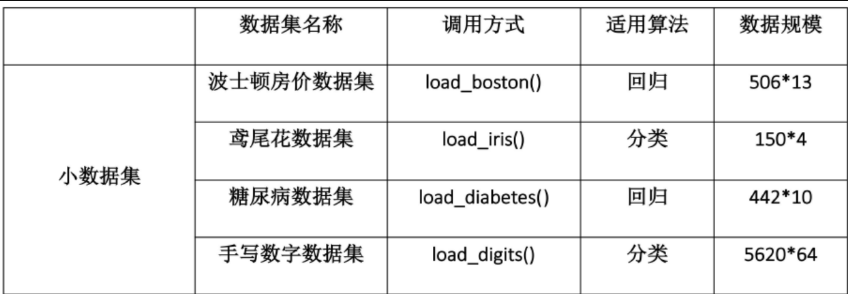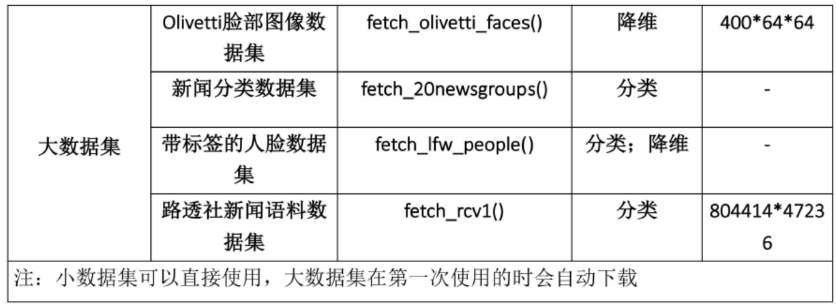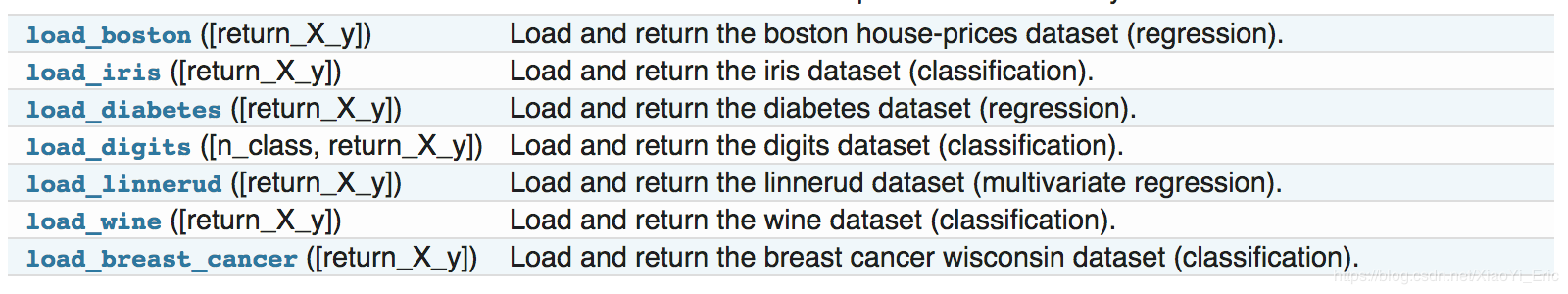这里我们使用iris的数据来举个例子，表示导出数据集：

iris = datasets.load_iris() # 导入数据集
X = iris.data # 获得其特征向量
y = iris.target # 获得样本label


注意，在0.18版本后，新增了一个功能：return_X_y=False

这个参数什么意思呢？就是控制输出数据的结构，若选为TRUE，则将因变量和自变量独立导出，我们看例子：

from sklearn.datasets import load_iris

print(X.shape, y.shape, type(X))
print(type(data))
# (150, 4) (150,) <class 'numpy.ndarray'>
# <class 'sklearn.utils.Bunch'>


简单来说，return_X_y 为TRUE，就是更方便了。

#### 1.1.1 　手写数字数据集

手写数字数据集包含1797个0-9的手写数字数据，每个数据由8 * 8 大小的矩阵构成，矩阵中值的范围是0-16，代表颜色的深度。

from sklearn.datasets import load_digits
print(digits.data.shape)
print(digits.target.shape)
print(digits.images.shape)
'''
(1797, 64)
(1797,)
(1797, 8, 8)
'''


展示如下：

import matplotlib.pyplot as plt

plt.matshow(digits.images)
plt.show()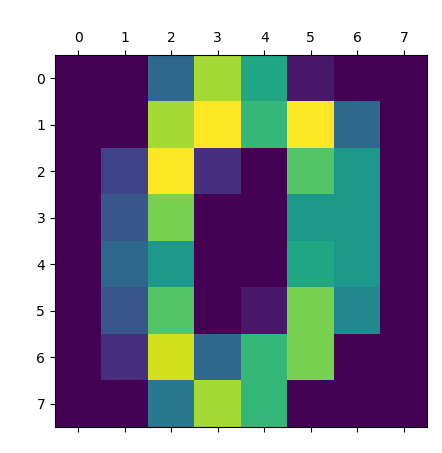#### 1.2 创建数据集

我们除了可以使用sklearn自带的数据集，还可以自己去创建训练样本，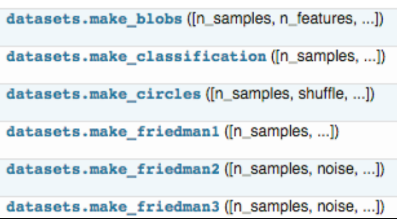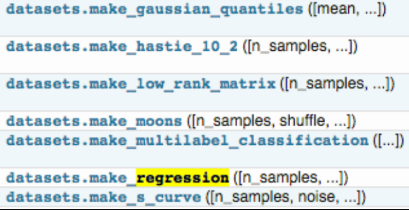下面我们拿分类问题的样本生成器举例子：

from sklearn.datasets.samples_generator import make_classification

X, y = make_classification(n_samples=6, n_features=5, n_informative=2,
n_redundant=2, n_classes=2, n_clusters_per_class=2, scale=1.0,
random_state=20)

# n_samples：指定样本数
# n_features：指定特征数
# n_classes：指定几分类
# random_state：随机种子，使得随机状可重


测试如下：

>>> for x_,y_ in zip(X,y):
print(y_,end=': ')
print(x_)

0: [-0.6600737  -0.0558978   0.82286793  1.1003977  -0.93493796]
1: [ 0.4113583   0.06249216 -0.90760075 -1.41296696  2.059838  ]
1: [ 1.52452016 -0.01867812  0.20900899  1.34422289 -1.61299022]
0: [-1.25725859  0.02347952 -0.28764782 -1.32091378 -0.88549315]
0: [-3.28323172  0.03899168 -0.43251277 -2.86249859 -1.10457948]
1: [ 1.68841011  0.06754955 -1.02805579 -0.83132182  0.93286635]


#### 1.2.1  用sklearn.datasets.make_blobs来生成数据

scikit中的make_blobs方法常被用来生成聚类算法的测试数据，直观地说，make_blobs会根据用户指定的特征数量，中心点数量，范围等来生成几类数据，这些数据可用于测试聚类算法的效果。

sklearn.datasets.make_blobs(n_samples=100, n_features=2, centers=3,
cluster_std=1.0, center_box=(-10.0, 10.0), shuffle=True,
random_state=None)[source]


输入：

• n_samples表示产生多少个数据
• n_features表示数据是几维
• centers表示数据点中心，可以输入int数字，代表有多少个中心，也可以输入几个坐标（fixed center locations）
• cluster_std表示分布的标准差

返回值：

• X，[n_samples, n_features]形状的数组，代表产生的样本
• y，[n_samples]形状的数组，代表每个点的标签(类别)

#### 例子（生成三类数据用于聚类（100个样本，每个样本2个特征））：

from sklearn.datasets import make_blobs
from matplotlib import pyplot

data,label = make_blobs(n_samples=100,n_features=2,centers=5)

# 绘制样本显示
pyplot.scatter(data[:,0],data[:,1],c=label)
pyplot.show()


结果：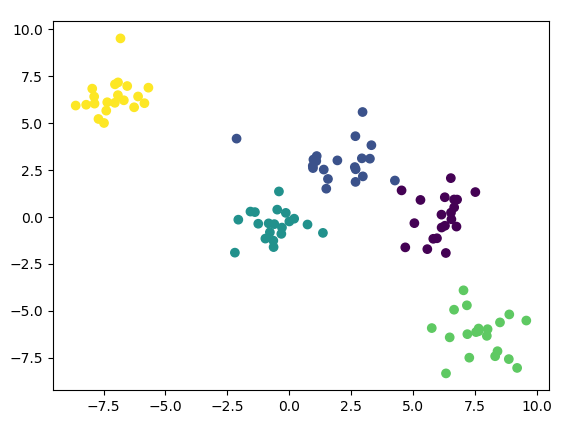#### 为每个类别设置不同的方差，只需要在上述代码中加入cluster_std参数即可：

import matplotlib.pylab as plt
from sklearn.datasets import make_blobs

# 每个样本有几个属性或者特征
n_features = 2

data,target = make_blobs(n_samples=100,n_features=2,centers=3,cluster_std=[1.0,2.0,3.0])
# 在2D图中绘制样本，每个样本颜色不同
plt.scatter(data[:,0],data[:,1],c=target)
plt.show()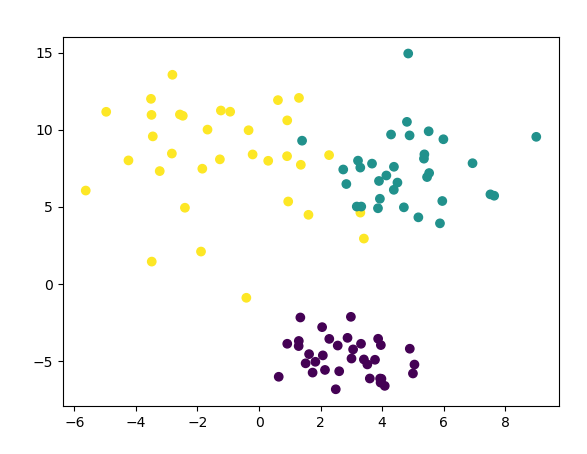#### 1.2.2  用sklearn.datasets.make_classification来生成数据

通常用于分类算法

sklearn.datasets.make_classification(n_samples=100, n_features=20,
n_informative=2, n_redundant=2,n_repeated=0, n_classes=2,
n_clusters_per_class=2, weights=None,flip_y=0.01, class_sep=1.0,
hypercube=True,shift=0.0, scale=1.0, shuffle=True, random_state=None)


输入：

1. n_features :特征个数= n_informative（） + n_redundant + n_repeated
2. n_informative：多信息特征的个数
3. n_redundant：冗余信息，informative特征的随机线性组合
4. n_repeated ：重复信息，随机提取n_informative和n_redundant 特征
5. n_classes：分类类别
6. n_clusters_per_class ：某一个类别是由几个cluster构成的

#### 1.2.3  用sklearn.datasets.make_gaussian和make_hastie_10_2来生成数据

sklearn.datasets.make_gaussian_quantiles(mean=None, cov=1.0, n_samples=100,
n_features=2, n_classes=3,shuffle=True, random_state=None)


利用高斯分位点区分不同数据

sklearn.datasets.make_hastie_10_2(n_samples=12000, random_state=None)


利用Hastie算法，生成二分类数据

import matplotlib.pyplot as plt

from sklearn.datasets import make_classification
from sklearn.datasets import make_blobs
from sklearn.datasets import make_gaussian_quantiles
from sklearn.datasets import make_hastie_10_2

plt.figure(figsize=(8, 8))

plt.subplot(421)
plt.title("One informative feature, one cluster per class", fontsize='small')
X1, Y1 = make_classification(n_samples=1000,n_features=2, n_redundant=0, n_informative=1,
n_clusters_per_class=1)
plt.scatter(X1[:, 0], X1[:, 1], marker='o', c=Y1)

plt.subplot(422)
plt.title("Two informative features, one cluster per class", fontsize='small')
X1, Y1 = make_classification(n_samples=1000,n_features=2, n_redundant=0, n_informative=2,
n_clusters_per_class=1)
plt.scatter(X1[:, 0], X1[:, 1], marker='o', c=Y1)

plt.subplot(423)
plt.title("Two informative features, two clusters per class", fontsize='small')
X2, Y2 = make_classification(n_samples=1000,n_features=2, n_redundant=0, n_informative=2)
plt.scatter(X2[:, 0], X2[:, 1], marker='o', c=Y2)

plt.subplot(424)
plt.title("Multi-class, two informative features, one cluster",
fontsize='small')
X1, Y1 = make_classification(n_samples=1000,n_features=2, n_redundant=0, n_informative=2,
n_clusters_per_class=1, n_classes=3)
plt.scatter(X1[:, 0], X1[:, 1], marker='o', c=Y1)

plt.subplot(425)
plt.title("Three blobs", fontsize='small')
X1, Y1 = make_blobs(n_samples=1000,n_features=2, centers=3)
plt.scatter(X1[:, 0], X1[:, 1], marker='o', c=Y1)

plt.subplot(426)
plt.title("Gaussian divided into four quantiles", fontsize='small')
X1, Y1 = make_gaussian_quantiles(n_samples=1000,n_features=2, n_classes=4)
plt.scatter(X1[:, 0], X1[:, 1], marker='o', c=Y1)

plt.subplot(427)
plt.title("hastie data ", fontsize='small')
X1, Y1 = make_hastie_10_2(n_samples=1000)
plt.scatter(X1[:, 0], X1[:, 1], marker='o', c=Y1)
plt.show()


结果：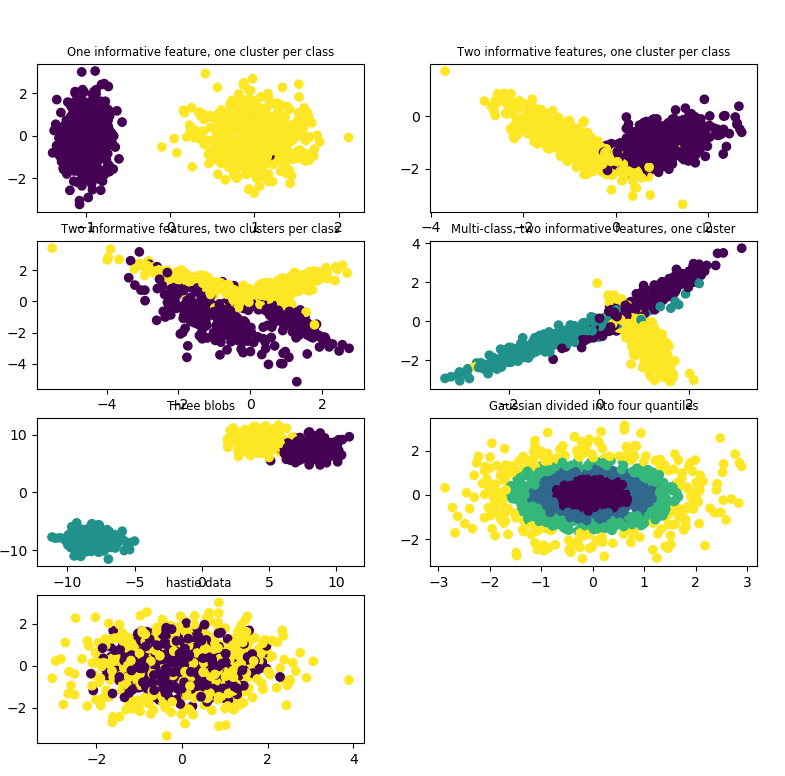#### 1.2.4  用sklearn.datasets.make_circles和make_moons来生成数据

生成环线数据

sklearn.datasets.make_circles(n_samples=100, shuffle=True, noise=None,
random_state=None, factor=0.8)


factor：外环和内环的尺度因子<1

sklearn.datasets.make_moons(n_samples=100, shuffle=True, noise=None,
random_state=None)


生成半环图

from sklearn.datasets import make_circles
from sklearn.datasets import make_moons
import matplotlib.pyplot as plt
import numpy as np

fig=plt.figure(1)
x1,y1=make_circles(n_samples=1000,factor=0.5,noise=0.1)
plt.subplot(121)
plt.title('make_circles function example')
plt.scatter(x1[:,0],x1[:,1],marker='o',c=y1)

plt.subplot(122)
x1,y1=make_moons(n_samples=1000,noise=0.1)
plt.title('make_moons function example')
plt.scatter(x1[:,0],x1[:,1],marker='o',c=y1)
plt.show()


结果：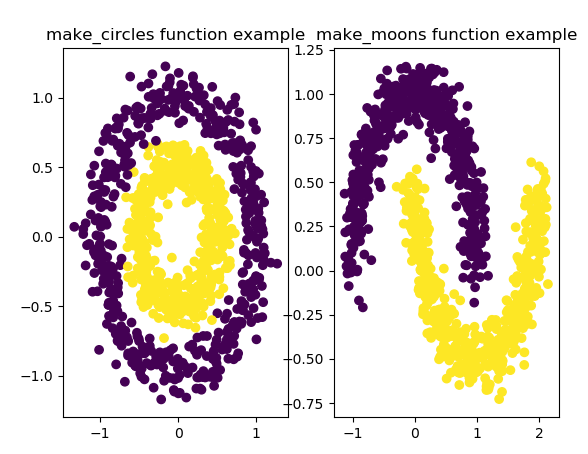### 2，数据预处理

数据预处理阶段是机器学习中不可缺少的一环，它会使得数据更加有效的被模型或者评估器识别。下面我们来看一下sklearn中有哪些平时我们常用的函数：

from sklearn import preprocessing


为了使得训练数据的标准化规则与测试数据的标准化规则同步，preprocessing中提供了很多的Scaler：

• StandardScaler
• MaxAbsScaler
• MinMaxScaler
• RobustScaler
• Normalizer
• 等其他预处理操作

对应的有直接的函数使用：scale()，maxabs_scale()，minmax_scale()，robust_scale()，normaizer（）

sklearn.preprocessing.scale(X)


#### 2.1 数据标准化

标准化：在机器学习中，我们可能要处理不同种类的资料，例如，音讯和图片上的像素值，这些资料可能是高纬度的，资料标准化后会使得每个特征中的数值平均变为0（将每个特征的值都减掉原始资料中该特征的平均），标准差变为1，这个方法被广泛的使用在许多机器学习算法中（例如：支持向量机，逻辑回归和类神经网络）。

StandardScaler计算训练集的平均值和标准差，以便测试数据及使用相同的变换。

变换后各维特征有0均值，单位方差，也叫z-score规范化（零均值规范化），计算方式是将特征值减去均值，除以标准差。

fit

用于计算训练数据的均值和方差，后面就会用均值和方差来转换训练数据

fit_transform

不仅计算训练数据的均值和方差，还会基于计算出来的均值和方差来转换训练数据，从而把数据转化成标准的正态分布。

transform

很显然，它只是进行转换，只是把训练数据转换成标准的正态分布。（一般会把train和test集放在一起做标准化，或者在train集上做标准化后，用同样的标准化器去标准化test集，此时可以使用scaler)。

data = [[0, 0], [0, 0], [1, 1], [1, 1]]
# 1. 基于mean和std的标准化
scaler = preprocessing.StandardScaler().fit(train_data)
scaler.transform(train_data)
scaler.transform(test_data)



一般来说先使用fit：

scaler = preocessing.StandardScaler().fit(X)


这一步可以计算得到scaler，scaler里面存的有计算出来的均值和方差。

再使用transform

scaler.transform(X)


这一步再用scaler中的均值和方差来转换X，使X标准化。

最后，在预测的时候，也要对数据做同样的标准化处理，即也要用上面的scaler中的均值和方差来对预测时候的特征进行标准化。

注意：测试数据和预测数据的标准化的方式要和训练数据标准化的方式一样，必须使用同一个scaler来进行transform

#### 2.2 最小-最大规范化

最小最大规范化对原始数据进行线性变换，变换到[0,1]区间（也可以是其他固定最小最大值的区间）。

# 2. 将每个特征值归一化到一个固定范围
scaler = preprocessing.MinMaxScaler(feature_range=(0, 1)).fit(train_data)
scaler.transform(train_data)
scaler.transform(test_data)
#feature_range: 定义归一化范围，注用（）括起来


#### 2.3 正则化（normalize）

当你想要计算两个样本的相似度时必不可少的一个操作，就是正则化。其思想是：首先求出样本的p范数，然后该样本的所有元素都要除以该范数，这样最终使得每个样本的范数都是1。规范化（Normalization）是将不同变化范围的值映射到相同的固定范围，常见的是[0,1]，也成为归一化。

如下例子，将每个样本变换成unit norm。

>>> X = [[ 1., -1.,  2.],
...      [ 2.,  0.,  0.],
...      [ 0.,  1., -1.]]
>>> X_normalized = preprocessing.normalize(X, norm='l2')

>>> X_normalized
array([[ 0.40..., -0.40...,  0.81...],
[ 1.  ...,  0.  ...,  0.  ...],
[ 0.  ...,  0.70..., -0.70...]])


我们可以发现对于每一个样本都有0.4^2+0.4^2+0.81^2=1。这就是L2 norm，变换后每个样本的各维特征的平方和为1.类似的，L1 norm则是变换后每个样本的各维特征的绝对值之和为1.还有max norm，则是将每个样本的各维特征除以该样本各维特征的最大值，

在度量样本之间相似性时，如果使用的是二次型kernel，则需要做Normalization。

#### 2.4 one-hot编码

one-hot编码是一种对离散特征值的编码方式，在LR模型中常用到，用于给线性模型增加非线性能力。

data = [[0, 0, 3], [1, 1, 0], [0, 2, 1], [1, 0, 2]]
encoder = preprocessing.OneHotEncoder().fit(data)
enc.transform(data).toarray()


#### 2.5 特征二值化（Binarization）

给定阈值，将特征转换为0/1.

binarizer = sklearn.preprocessing.Binarizer(threshold=1.1)

binarizer.transform(X)


#### 2.6 类别特征编码

有时候特征时类别型的，而一些算法的输入必须是数值型，此时需要对其编码，

enc = preprocessing.OneHotEncoder()
enc.fit([[0, 0, 3], [1, 1, 0], [0, 2, 1], [1, 0, 2]])
enc.transform([[0, 1, 3]]).toarray()  #array([[ 1., 0., 0., 1., 0., 0., 0., 0., 1.]])


上面这个例子，第一维特征有两种值0和1，用两位去编码。第二维用三位，第三维用四位。

#### 2.7 标签编码（Label encoding）

le = sklearn.preprocessing.LabelEncoder()
le.fit([1, 2, 2, 6])
le.transform([1, 1, 2, 6])  #array([0, 0, 1, 2])
#非数值型转化为数值型
le.fit(["paris", "paris", "tokyo", "amsterdam"])
le.transform(["tokyo", "tokyo", "paris"])  #array([2, 2, 1])


### 3，数据集拆分

在得到训练数据集时，通常我们经常会把训练数据进一步拆分成训练集和验证集，这样有助于我们模型参数的选取。

train_test_split是交叉验证中常用的函数，功能是从样本中随机的按比例选取train datatestdata，形式为：

X_train,X_test, y_train, y_test =

cross_validation.train_test_split(train_data,train_target,test_size=0.4, random_state=0)


注意：train_test_split 不再 cross_validation中，已经移到 model_selection 中。

#### 参数解释

• train_data：所要划分的样本特征集
• train_target：所要划分的样本结果
• test_size：样本占比，如果是整数的话就是样本的数量
• random_state：是随机数的种子。
• 随机数种子：其实就是该组随机数的编号，在需要重复试验的时候，保证得到一组一样的随机数。比如你每次都填1其他参数一样的情况下你得到的随机数组是一样的。但填0或不填，每次都会不一样。
• 随机数的产生取决于种子，随机数和种子之间的关系遵从以下两个规则：

种子不同，产生不同的随机数

种子相同，即使实例不同也产生相同的随机数

#### 参数说明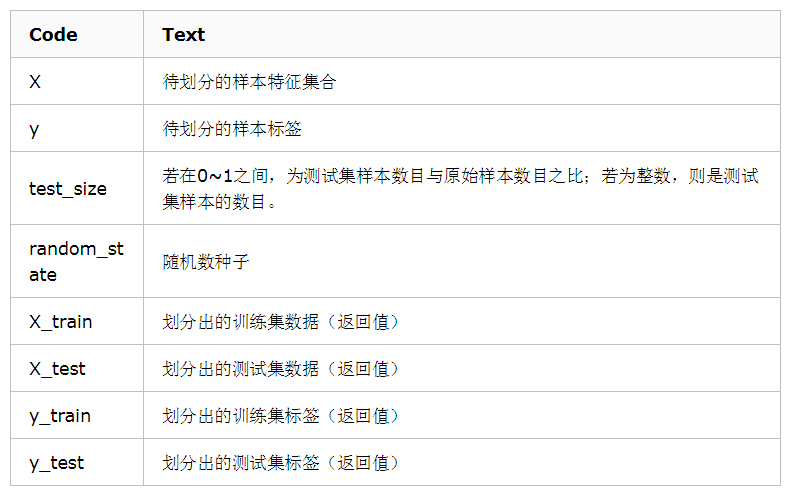#### 示例

# 作用：将数据集划分为 训练集和测试集
# 格式：train_test_split(*arrays, **options)
from sklearn.mode_selection import train_test_split

X_train, X_test, y_train, y_test = train_test_split(X, y, test_size=0.3, random_state=42)
"""

---
arrays：样本数组，包含特征向量和标签

test_size：
float-获得多大比重的测试样本 （默认：0.25）
int - 获得多少个测试样本

train_size: 同test_size

random_state:
int - 随机种子（种子固定，实验可复现）

shuffle - 是否在分割之前对数据进行洗牌（默认True）

---

(train-test split)
"""


#### 拆分参数遇到的问题及其解决方法

导入模块

from sklearn.cross_validation import cross_val_score


则会报错，代码如下：

    from sklearn.cross_validation import cross_val_score
ModuleNotFoundError: No module named 'sklearn.cross_validation'


解决方法：

from sklearn.model_selection import cross_val_score


### 4，定义模型

在这一步我们首先要分析自己数据的类型，明白自己要用什么模型来做，然后我们就可以在sklearn中定义模型了，sklearn为所有模型提供了非常相似的接口，这样使得我们可以更加快速的熟悉所有模型的用法，在这之前，我们先来看看模型的常用属性和功能。

# 拟合模型
model.fit(X_train, y_train)
# 模型预测
model.predict(X_test)

# 获得这个模型的参数
model.get_params()
# 为模型进行打分
model.score(data_X, data_y) # 线性回归：R square； 分类问题： acc


#### 4.1 线性回归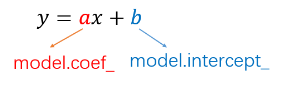from sklearn.linear_model import LinearRegression
# 定义线性回归模型
model = LinearRegression(fit_intercept=True, normalize=False,
copy_X=True, n_jobs=1)

"""

---
fit_intercept：是否计算截距。False-模型没有截距
normalize： 当fit_intercept设置为False时，该参数将被忽略。 如果为真，

n_jobs：指定线程数
"""


#### 4.2 逻辑回归LR

from sklearn.linear_model import LogisticRegression
# 定义逻辑回归模型
model = LogisticRegression(penalty=’l2’, dual=False, tol=0.0001, C=1.0,
fit_intercept=True, intercept_scaling=1, class_weight=None,
random_state=None, solver=’liblinear’, max_iter=100, multi_class=’ovr’,
verbose=0, warm_start=False, n_jobs=1)

"""参数
---
penalty：使用指定正则化项（默认：l2）
dual: n_samples > n_features取False（默认）
C：正则化强度的反，值越小正则化强度越大
n_jobs: 指定线程数
random_state：随机数生成器
fit_intercept: 是否需要常量
"""


#### 4.3 朴素贝叶斯算法NB（Naive Bayes）

from sklearn import naive_bayes
model = naive_bayes.GaussianNB() # 高斯贝叶斯
model = naive_bayes.MultinomialNB(alpha=1.0, fit_prior=True, class_prior=None)
model = naive_bayes.BernoulliNB(alpha=1.0, binarize=0.0, fit_prior=True, class_prior=None)
"""

---
alpha：平滑参数
fit_prior：是否要学习类的先验概率；false-使用统一的先验概率
class_prior: 是否指定类的先验概率；若指定则不能根据参数调整
binarize: 二值化的阈值，若为None，则假设输入由二进制向量组成
"""


#### 4.4 决策树DT

from sklearn import tree
model = tree.DecisionTreeClassifier(criterion=’gini’, max_depth=None,
min_samples_split=2, min_samples_leaf=1, min_weight_fraction_leaf=0.0,
max_features=None, random_state=None, max_leaf_nodes=None,
min_impurity_decrease=0.0, min_impurity_split=None,
class_weight=None, presort=False)
"""参数
---
criterion ：特征选择准则gini/entropy
max_depth：树的最大深度，None-尽量下分
min_samples_split：分裂内部节点，所需要的最小样本树
min_samples_leaf：叶子节点所需要的最小样本数
max_features: 寻找最优分割点时的最大特征数
max_leaf_nodes：优先增长到最大叶子节点数
min_impurity_decrease：如果这种分离导致杂质的减少大于或等于这个值，则节点将被拆分。
"""


#### 4.5 支持向量机SVM

from sklearn.svm import SVC
model = SVC(C=1.0, kernel=’rbf’, gamma=’auto’)
"""参数
---
C：误差项的惩罚参数C
gamma: 核相关系数。浮点数，If gamma is ‘auto’ then 1/n_features will be used instead.
"""


#### 4.6 k近邻算法KNN

from sklearn import neighbors
#定义kNN分类模型
model = neighbors.KNeighborsClassifier(n_neighbors=5, n_jobs=1) # 分类
model = neighbors.KNeighborsRegressor(n_neighbors=5, n_jobs=1) # 回归
"""参数
---
n_neighbors： 使用邻居的数目
n_jobs：并行任务数
"""


#### 4.7 多层感知器（神经网络）

from sklearn.neural_network import MLPClassifier
# 定义多层感知机分类算法
"""参数
---
hidden_layer_sizes: 元祖
activation：激活函数
alpha：L2惩罚(正则化项)参数。
"""


### 5，模型评估与选择

评价指标针对不同的机器学习任务有不同的指标，同一任务也有不同侧重点的评价指标。以下方法，sklearn中都在sklearn.metrics类下，务必记住那些指标适合分类，那些适合回归。

机器学习常用的评估指标请参考博文：Python机器学习笔记：常用评估指标的前世今生

#### 5.1 交叉验证

• 分类：accuracy(准确率)、f1、f1_micro、f1_macro（这两个用于多分类的f1_score）、precision(精确度)、recall(召回率)、roc_auc
• 回归：neg_mean_squared_error（MSE、均方误差）、r2

from sklearn.model_selection import cross_val_score
cross_val_score(model, X, y=None, scoring=None, cv=None, n_jobs=1)
"""参数
---
model：拟合数据的模型
cv ： k-fold
scoring: 打分参数-‘accuracy’、‘f1’、‘precision’、‘recall’ 、‘roc_auc’、'neg_log_loss'等等
"""


### （补充）：交叉验证的学习

#### 1，导入k折交叉验证模块

注意cross_val_score 是根据模型进行计算，计算交叉验证的结果，可以简单的认为cross_val_score中调用了KFold 进行数据集划分。

from sklearn.model_selection import cross_val_score


#### 2，交叉验证的思想

把某种意义下将原始数据（dataset）进行分组，一部分作为训练集（train set），另一部分作为验证集（validation set or test set），首先用训练集对分类器进行训练，再利用验证集来测试训练得到的模型（model），以此来作为评价分类器的性能指标。

#### 3，为什么使用交叉验证法

• 交叉验证用于评估模型的预测性能，尤其是训练好的模型在新数据上的表现，可以在一定程序熵减少过拟合。
• 交叉验证还可以从有限的数据中获取尽可能多的有效信息

#### 4，model_selection.KFold 和 model_selection.cross_val_score的区别

我们直接看官网：KFold：https://scikit-learn.org/stable/modules/generated/sklearn.model_selection.KFold.html#sklearn.model_selection.KFold

cross_val_score：https://scikit-learn.org/stable/modules/generated/sklearn.model_selection.cross_val_score.html#sklearn.model_selection.cross_val_score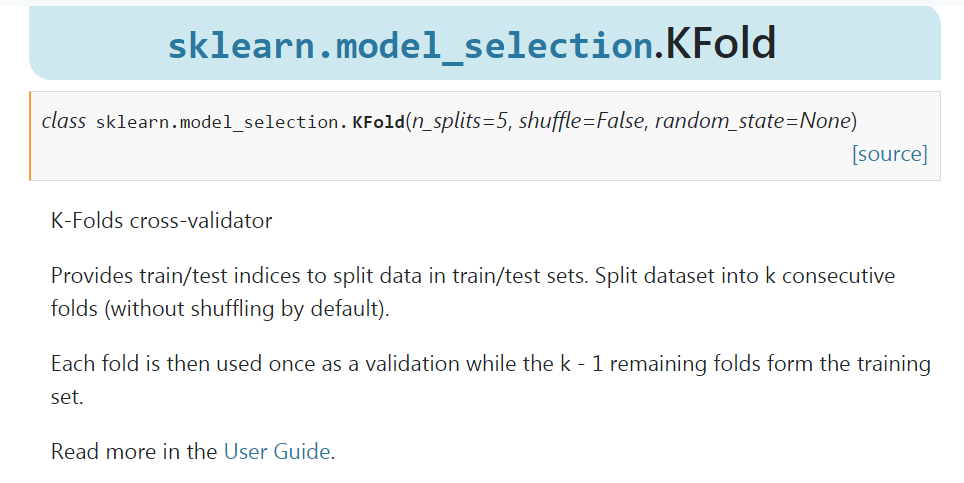KFold 就是对数据集划分为 训练集/测试集，然后将训练数据集划分为K折，每个折进行一次验证，而剩下的K-1 折进行训练，依次循环，直到用完所有的折。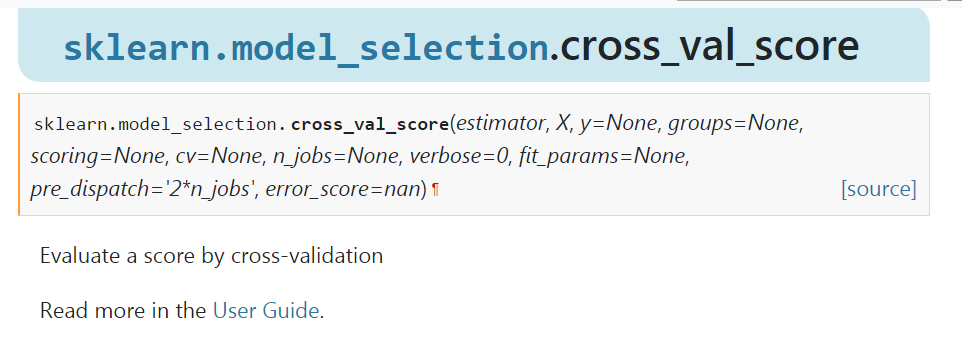而 cross_val_score 就是通过交叉验证评估得分

下面看看K折交叉验证函数KFold函数：

KFold（n_split, shuffle, random_state）

参数：n_split:要划分的折数

shuffle: 每次都进行shuffle，测试集中折数的总和就是训练集的个数

random_state:随机状态


其使用如下：

from sklearn.model_selection import KFold
from sklearn.linear_model import LinearRegression
import numpy as np
import pandas as pd

X = np.array([[1, 2], [3, 4], [1, 2], [3, 4]])
y = np.array([1, 2, 3, 4])
kf = KFold(n_splits=2)
# get_n_splits: Returns the number of splitting iterations in the cross-validator
print(kf.get_n_splits(X))  # 2

KF = KFold(n_splits=5)
alg = LinearRegression()
# 这里想强行使用DataFrame的数据格式，因为以后大家读取数据使用都是csv格式
# 所以必不可免要用 iloc
X, Y = pd.DataFrame(X), pd.DataFrame(Y)
# split()：Generate indices to split data into training and test set.
for train_index, test_index in KF.split(X):
print("TRAIN:", train_index, "TEST:", test_index)
X_train, X_test = X.iloc[train_index], X.iloc[test_index]
y_train, y_test = Y.iloc[train_index], Y.iloc[test_index]
alg.fit(X_train, y_train)
# 后面就自由发挥即可


#### 5，主要有哪些方法

1，留出法（holdout cross validation）

在机器学习任务中，拿到数据后，我们首先会将原始数据集分为三部分：训练集，验证集和测试集。

训练集用于训练模型，验证集用于模型的参数选择配置，测试集对于模型来说是未知数据，用于评估模型的泛化能力。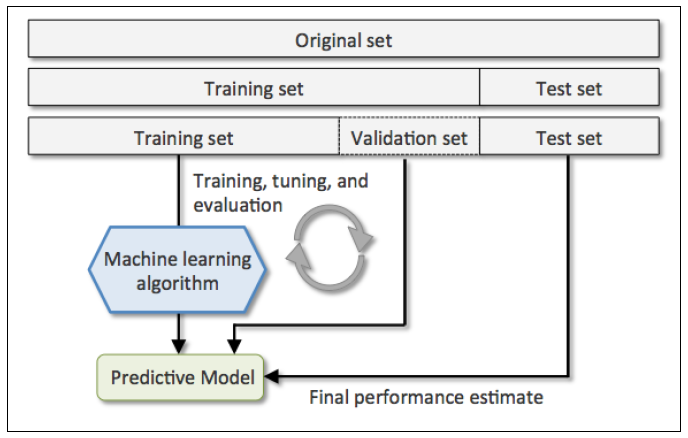这个方法操作简单，只需要随机将原始数据分为三组即可。

不过如果只做一次分割，它对训练集，验证集和测试机的样本比例，还有分割后数据的分布是否和原始数据集的分布相同等因素比较敏感，不同的划分会得到不同的最优模型，，而且分成三个集合后，用于训练的数据更少了。于是又了2.k折交叉验证（k-fold cross validation）.

下面例子，一共有150条数据：

>>> import numpy as np
>>> from sklearn.model_selection import train_test_split
>>> from sklearn import datasets
>>> from sklearn import svm

>>> iris.data.shape, iris.target.shape
((150, 4), (150,))


用train_test_split来随机划分数据集，其中40%用于测试集，有60条数据，60%为训练集，有90条数据：

>>> X_train, X_test, y_train, y_test = train_test_split(
...     iris.data, iris.target, test_size=0.4, random_state=0)

>>> X_train.shape, y_train.shape
((90, 4), (90,))
>>> X_test.shape, y_test.shape
((60, 4), (60,))


用train来训练，用test来评价模型的分数。

>>> clf = svm.SVC(kernel='linear', C=1).fit(X_train, y_train)
>>> clf.score(X_test, y_test)
0.96...


2，2. k 折交叉验证（k-fold cross validation）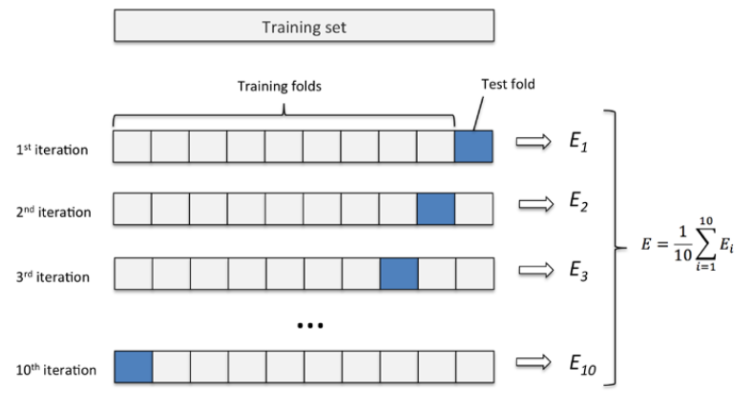K折交叉验证通过对k个不同分组训练的结果进行平均来减少方差，因此模型的性能对数据的划分就不那么敏感。

• 第一步，不重复抽样将原始数据随机分为 k 份。
• 第二步，每一次挑选其中 1 份作为测试集，剩余 k-1 份作为训练集用于模型训练。
• 第三步，重复第二步 k 次，这样每个子集都有一次机会作为测试集，其余机会作为训练集。
• 在每个训练集上训练后得到一个模型，
• 用这个模型在相应的测试集上测试，计算并保存模型的评估指标，
• 第四步，计算 k 组测试结果的平均值作为模型精度的估计，并作为当前 k 折交叉验证下模型的性能指标。

K一般取10，数据量小的是，k可以设大一点，这样训练集占整体比例就比较大，不过同时训练的模型个数也增多。数据量大的时候，k可以设置小一点。当k=m的时候，即样本总数，出现了留一法。

举例，这里直接调用了cross_val_score，这里用了5折交叉验证

>>> from sklearn.model_selection import cross_val_score
>>> clf = svm.SVC(kernel='linear', C=1)
>>> scores = cross_val_score(clf, iris.data, iris.target, cv=5)
>>> scores
array([ 0.96...,  1.  ...,  0.96...,  0.96...,  1.        ])


得到最后平均分数为0.98，以及它的95%置信区间：

>>> print("Accuracy: %0.2f (+/- %0.2f)" % (scores.mean(), scores.std() * 2))
Accuracy: 0.98 (+/- 0.03)


我们可以直接看一下K-Fold是怎么样划分数据的：X有四个数据，把它分成2折，结构中最后一个集合是测试集，前面的是训练集，每一行为1折：

>>> import numpy as np
>>> from sklearn.model_selection import KFold

>>> X = ["a", "b", "c", "d"]
>>> kf = KFold(n_splits=2)
>>> for train, test in kf.split(X):
...     print("%s %s" % (train, test))
[2 3] [0 1]
[0 1] [2 3]


同样的数据X，我们来看LeaveOneOut后是什么样子，那就是把它分成4折，结果中最后一个集合是测试集，只有一个元素，前面的是训练集，每一行为1折：

>>> from sklearn.model_selection import LeaveOneOut

>>> X = [1, 2, 3, 4]
>>> loo = LeaveOneOut()
>>> for train, test in loo.split(X):
...     print("%s %s" % (train, test))
[1 2 3] 
[0 2 3] 
[0 1 3] 
[0 1 2] 


3，留一法（Leave one out cross validation）

每次的测试集都只有一个样本，要进行m次训练和预测，这个方法用于训练的数据只比整体数据集少一个样本，因此最接近原始样本的分布。但是训练复杂度增加了，因为模型的数量与原始数据样本数量相同。一般在数据缺少时使用。

• 多次 k 折交叉验证再求均值，例如：10 次 10 折交叉验证，以求更精确一点。
• 划分时有多种方法，例如对非平衡数据可以用分层采样，就是在每一份子集中都保持和原始数据集相同的类别比例。
• 模型训练过程的所有步骤，包括模型选择，特征选择等都是在单个折叠 fold 中独立执行的。

4，Bootstrapping

通过自助采样法，即在含有 m 个样本的数据集中，每次随机挑选一个样本，再放回到数据集中，再随机挑选一个样本，这样有放回地进行抽样 m 次，组成了新的数据集作为训练集。

这里会有重复多次的样本，也会有一次都没有出现的样本，原数据集中大概有 36.8% 的样本不会出现在新组数据集中。

优点是训练集的样本总数和原数据集一样都是 m，并且仍有约 1/3 的数据不被训练而可以作为测试集。
缺点是这样产生的训练集的数据分布和原数据集的不一样了，会引入估计偏差。
（此种方法不是很常用，除非数据量真的很少）

#### 5.2 检验曲线

使用检验曲线，我们可以更加方便的改变模型参数，获取模型表现。

from sklearn.model_selection import validation_curve
train_score, test_score = validation_curve(model, X, y, param_name, param_range, cv=None, scoring=None, n_jobs=1)
"""参数
---
model:用于fit和predict的对象
X, y: 训练集的特征和标签
param_name：将被改变的参数的名字
param_range： 参数的改变范围
cv：k-fold

---
train_score: 训练集得分（array）
test_score: 验证集得分（array）
"""


#### 5.3 分类模型

• accuracy_score（准确率得分）是模型分类正确的数据除以样本总数 【模型的score方法算的也是准确率】
accuracy_score(y_test,y_pre)
# 或者 model.score(x_test,y_test)，大多模型都是有score方法的


• classification_report中的各项得分的avg/total 是每一分类占总数的比例加权算出来的
print(classification_report(y_test,y_log_pre))

precision    recall  f1-score   support

0       0.87      0.94      0.90       105
1       0.91      0.79      0.85        73

avg / total       0.88      0.88      0.88       178


• confusion_matrix（混淆矩阵），用来评估分类的准确性
>>> from sklearn.metrics import confusion_matrix
>>> y_true = [2, 0, 2, 2, 0, 1]
>>> y_pred = [0, 0, 2, 2, 0, 2]
>>> confusion_matrix(y_true, y_pred)
array([[2, 0, 0],
[0, 0, 1],
[1, 0, 2]])


• precision_score(精确度)、recall_score(召回率)、f1_score（后者由前两个推导出的）
这三个不仅适合二分类，也适合多分类。只需要指出参数average=‘micro’/‘macro’/'weighted’

macro：计算二分类metrics的均值，为每个类给出相同权重的分值。

micro： 给出了每个样本类以及它对整个metrics的贡献的pair（sample-
weight），而非对整个类的metrics求和，它会每个类的metrics上的权重及

weighted: 对于不均衡数量的类来说，计算二分类metrics的平均，


• roc_curve（ROC曲线，用于二分类）

### 6 保存模型

最后，我们可以将我们训练好的model保存到本地，或者放到线上供用户使用，那么如何保存训练好的model呢？主要有下面两种方式：

#### 6.1 保存为pickle文件

import pickle

# 保存模型
with open('model.pickle', 'wb') as f:
pickle.dump(model, f)

# 读取模型
with open('model.pickle', 'rb') as f:
model.predict(X_test)


#### 6.2 sklearn自带方法joblib

from sklearn.externals import joblib

# 保存模型
joblib.dump(model, 'model.pickle')

#载入模型


### 7，模型评分

1，模型的score方法：最简单的模型评估方法就是调用模型自己的方法：

# 预测
y_predict = knnClf.predict(x_test)
print("score on the testdata:",knnClf.score(x_test,y_test))


2，sklearn的指标函数：库提供的一些计算方法，常用的有classification_report方法

3，sklearn也支持自己开发评价方法。

### 8，几种交叉验证（cross validation）方式的比较

模型评价的目的：通过模型评价，我们知道当前训练模型的好坏，泛化能力如何？从而知道是否可以应用在解决问题上，如果不行，那又是那些出了问题？

#### train_test_split

在分类问题中，我们通常通过对训练集进行triain_test_split，划分出train 和test两部分，其中train用来训练模型，test用来评估模型，模型通过fit方法从train数据集中学习，然后调用score方法在test集上进行评估，打分；从分数上我们知道模型当前的训练水平如何。

from sklearn.datasets import load_breast_cancer
from sklearn.model_selection import train_test_split
from sklearn.linear_model import LogisticRegression
import  matplotlib.pyplot as plt

X_train,X_test,y_train,y_test = train_test_split(cancer.data,cancer.target,random_state=0)

logreg = LogisticRegression().fit(X_train,y_train)
print("Test set score:{:.2f}".format(logreg.score(X_test,y_test)))


结果：

Test set score:0.96


然而这这方式只进行了一次划分，数据结果具有偶然性，如果在某次划分中，训练集里全是容易学习的数据，测试集里全是复杂的数据，这样的就会导致最终的结果不尽人意。

#### Standard Cross Validation

针对上面通过train_test_split划分，从而进行模型评估方式存在的弊端，提出Cross Validation交叉验证。

Cross Validation：进行多次train_test_split划分；每次划分时，在不同的数据集上进行训练，测试评估，从而得到一个评价结果；如果是5折交叉验证，意思就是在原始数据集上，进行五次划分，每次划分进行一次训练，评估，最后得到5次划分后的评估结果，一般在这几次评估结果上取平均得到最后的评分，k-folf cross-validation ，其中K一般取5或10。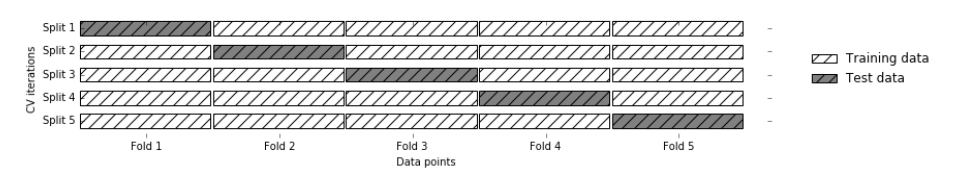代码：

from sklearn.model_selection import cross_val_score
from sklearn.model_selection import train_test_split
from sklearn.linear_model import LogisticRegression
import  warnings

warnings.filterwarnings('ignore')

X_train, X_test, y_train, y_test = train_test_split(
cancer.data , cancer.target, random_state=0
)

logreg = LogisticRegression()
# CV 默认是3折交叉验证，可以修改cv=5，变为5折交叉验证
scores = cross_val_score(logreg,cancer.data , cancer.target)

print("Cross validation scores:{}".format(scores))
print("Mean cross validation score:{:2f}".format(scores.mean()))


结果：

Cross validation scores:[0.93684211 0.96842105 0.94179894]
Mean cross validation score:0.949021


#### 交叉验证的优点：

• 原始采用的train_test_split方法，数据划分具有偶然性；交叉验证通过多次划分，大大降低了这种由一次随机划分带来的偶然性，同时通过多次划分，多次训练，模型也能遇到各种各样的数据，从而提高其泛化能力
• 与原始的train_test_split相比，对数据的使用效率更高，train_test_split，默认训练集，测试集比例为3:1，而对交叉验证来说，如果是5折交叉验证，训练集比测试集为4:1；10折交叉验证训练集比测试集为9:1.数据量越大，模型准确率越高！

#### 交叉验证的缺点：

这种简答的交叉验证方式，从上面的图片可以看出来，每次划分时对数据进行均分，设想一下，会不会存在一种情况：数据集有5类，抽取出来的也正好是按照类别划分的5类，也就是说第一折全是0类，第二折全是1类，等等；这样的结果就会导致，模型训练时。没有学习到测试集中数据的特点，从而导致模型得分很低，甚至为0，为避免这种情况，又出现了其他的各种交叉验证方式。

#### Stratifid k-fold cross validation

分层交叉验证（Stratified k-fold cross validation）：首先它属于交叉验证类型，分层的意思是说在每一折中都保持着原始数据中各个类别的比例关系，比如说：原始数据有3类，比例为1:2:1，采用3折分层交叉验证，那么划分的3折中，每一折中的数据类别保持着1:2:1的比例，这样的验证结果更加可信。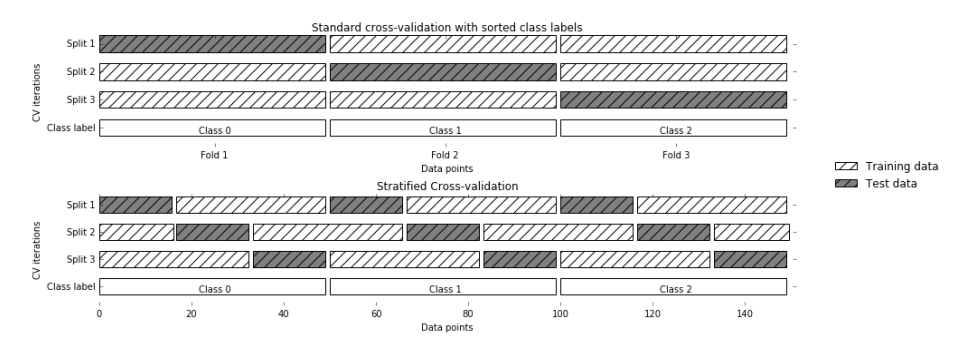from sklearn.datasets import load_iris
from sklearn.model_selection import StratifiedKFold ,cross_val_score
from sklearn.linear_model import LogisticRegression
import warnings

warnings.filterwarnings('ignore')

logreg = LogisticRegression()
strKFold = StratifiedKFold(n_splits=3,shuffle=False,random_state=0)
scores = cross_val_score(logreg,iris_data.data,iris_data.target,cv=strKFold)
print("straitified cross validation scores:{}".format(scores))
print("Mean score of straitified cross validation:{:.2f}".format(scores.mean()))


straitified cross validation scores:[0.96078431 0.92156863 0.95833333]
Mean score of straitified cross validation:0.95


#### Leave-one-out Cross-validation 留一法

留一法Leave-one-out Cross-validation：是一种特殊的交叉验证方式。顾名思义，如果样本容量为n，则k=n，进行n折交叉验证，每次留下一个样本进行验证。主要针对小样本数据。

from sklearn.datasets import load_iris
from sklearn.model_selection import LeaveOneOut , cross_val_score
from sklearn.linear_model import LogisticRegression
import  warnings

warnings.filterwarnings('ignore')

logreg = LogisticRegression()
loout = LeaveOneOut()
scores = cross_val_score(logreg,iris.data,iris.target,cv=loout)
print("leave-one-out cross validation scores:{}".format(scores))
print("Mean score of leave-one-out cross validation:{:.2f}".format(scores.mean()))


leave-one-out cross validation scores:[1. 1. 1. 1. 1. 1. 1. 1. 1. 1. 1. 1. 1. 1. 1. 1. 1. 1. 1. 1. 1. 1. 1. 1.
1. 1. 1. 1. 1. 1. 1. 1. 1. 1. 1. 1. 1. 1. 1. 1. 1. 1. 1. 1. 1. 1. 1. 1.
1. 1. 1. 1. 1. 1. 1. 1. 1. 1. 1. 1. 1. 1. 1. 1. 1. 1. 0. 1. 1. 1. 0. 1.
1. 1. 1. 1. 1. 1. 1. 1. 1. 1. 1. 0. 0. 0. 1. 1. 1. 1. 1. 1. 1. 1. 1. 1.
1. 1. 1. 1. 1. 1. 1. 1. 1. 1. 1. 1. 1. 1. 1. 1. 1. 1. 1. 1. 1. 1. 1. 1.
1. 1. 1. 1. 1. 1. 1. 1. 1. 0. 1. 1. 1. 0. 1. 1. 1. 1. 1. 1. 1. 1. 1. 1.
1. 1. 1. 1. 1. 1.]
Mean score of leave-one-out cross validation:0.95


#### Shuffle-split cross-validation

控制更加灵活，可以控制划分迭代次数，每次划分测试集和训练集的比例（也就说：可以存在机不再训练集也不再测试集的情况）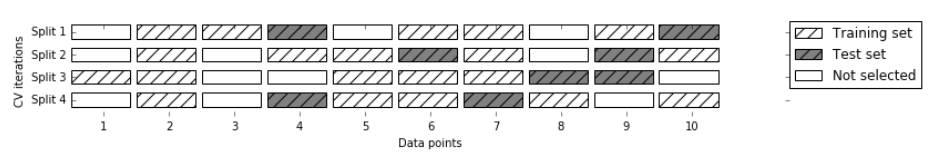from sklearn.datasets import load_iris
from sklearn.model_selection import ShuffleSplit,cross_val_score
from sklearn.linear_model import LogisticRegression
import warnings

warnings.filterwarnings('ignore')

# 迭代八次
shufsp1 = ShuffleSplit(train_size=0.5,test_size=0.4,n_splits=8)
logreg = LogisticRegression()
scores = cross_val_score(logreg,iris.data,iris.target,cv=shufsp1)

print("shuffle split cross validation scores:\n{}".format(scores))
print("Mean score of shuffle split cross validation:{:.2f}".format(scores.mean()))


shuffle split cross validation scores:
[0.95       1.         0.86666667 0.95       0.88333333 0.88333333
0.85       0.9       ]
Mean score of shuffle split cross validation:0.91


### 9，sklearn中一些函数的用法

#### 9.1  from sklearn.utils import shuffle 解析

在进行机器学习时，经常需要打乱样本，这种时候Python中第三方库提供了这个功能——sklearn.utils.shuffle。

#### 1，Parameters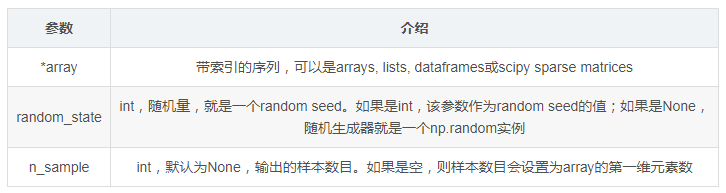#### 2，Returns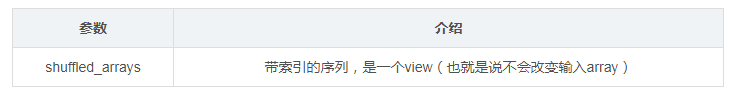https://www.cnblogs.com/magle/p/5638409.html

https://blog.csdn.net/u014248127/article/details/78885180

https://www.cnblogs.com/ysugyl/p/8707887.html

posted @ 2018-12-29 15:13  战争热诚  阅读(55681)  评论(6编辑  收藏